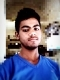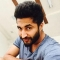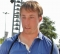## Graph ColoringUpdated on 16-Jun-2020 12:53:49

4K+ Views

Graph coloring problem is a special case of graph labeling. In this problem, each node is colored into some colors. But coloring has some constraints. We cannot use the same color for any adjacent vertices.For solving this problem, we need to use the greedy algorithm, but it does not guaranty to use minimum color.Input and OutputInput: Adjacency matrix of the graph. 0 0 1 0 1 0 0 1 1 1 1 1 0 1 0 0 1 1 0 1 1 1 0 1 0 Output: Node: 0, Assigned with Color: 0 Node: 1, Assigned with Color: 0 ... Read More

## Fleury’s AlgorithmUpdated on 16-Jun-2020 12:47:09

5K+ Views

Fleury’s Algorithm is used to display the Euler path or Euler circuit from a given graph. In this algorithm, starting from one edge, it tries to move other adjacent vertices by removing the previous vertices. Using this trick, the graph becomes simpler in each step to find the Euler path or circuit.We have to check some rules to get the path or circuit −The graph must be a Euler Graph.When there are two edges, one is bridge, another one is non-bridge, we have to choose non-bridge at first.Choosing of starting vertex is also tricky, we cannot use any vertex as ... Read More

## Eulerian Path and CircuitUpdated on 16-Jun-2020 13:20:51

5K+ Views

The Euler path is a path, by which we can visit every edge exactly once. We can use the same vertices for multiple times. The Euler Circuit is a special type of Euler path. When the starting vertex of the Euler path is also connected with the ending vertex of that path, then it is called the Euler Circuit.To detect the path and circuit, we have to follow these conditions −The graph must be connected.When exactly two vertices have odd degree, it is a Euler Path.Now when no vertices of an undirected graph have odd degree, then it is a ... Read More

## Euler Circuit in a Directed GraphUpdated on 16-Jun-2020 11:20:28

1K+ Views

The Euler path is a path, by which we can visit every edge exactly once. We can use the same vertices for multiple times. The Euler Circuit is a special type of Euler path. When the starting vertex of the Euler path is also connected with the ending vertex of that path, then it is called the Euler Circuit.To check whether a graph is Eulerian or not, we have to check two conditions −The graph must be connected.The in-degree and out-degree of each vertex must be the same.Input and OutputInput: Adjacency matrix of the graph. 0 1 0 0 0 ... Read More

## Detect Cycle in a Directed GraphUpdated on 16-Jun-2020 11:25:14

2K+ Views

Using a Depth First Search (DFS) traversal algorithm we can detect cycles in a directed graph. If there is any self-loop in any node, it will be considered as a cycle, otherwise, when the child node has another edge to connect its parent, it will also a cycle.For the disconnected graph, there may different trees present, we can call them a forest. Now we have to detect cycle for all trees of the forest.In this approach, we will use different sets to assign nodes to perform the DFS traversal. There are three different sets, the White, Grey and the Black. ... Read More

## Detect Cycle in a an Undirected GraphUpdated on 16-Jun-2020 11:27:25

6K+ Views

To detect if there is any cycle in the undirected graph or not, we will use the DFS traversal for the given graph. For every visited vertex v, when we have found any adjacent vertex u, such that u is already visited, and u is not the parent of vertex v. Then one cycle is detected. We will assume that there are no parallel edges for any pair of vertices.Input and Output: Adjacency matrix         0 1 0 0 0     1 0 1 1 0     0 1 0 0 1     0 1 ... Read More

## Depth First Search (DFS) for a GraphUpdated on 16-Jun-2020 11:31:14

2K+ Views

The Depth-First Search (DFS) is a graph traversal algorithm. In this algorithm, one starting vertex is given, and when an adjacent vertex is found, it moves to that adjacent vertex first and tries to traverse in the same manner.It moves through the whole depth, as much as it can go, after that it backtracks to reach previous vertices to find the new path.To implement DFS in an iterative way, we need to use the stack data structure. If we want to do it recursively, external stacks are not needed, it can be done internal stacks for the recursion calls.Input and ... Read More

## Connectivity in a directed graphUpdated on 16-Jun-2020 11:35:48

2K+ Views

To check connectivity of a graph, we will try to traverse all nodes using any traversal algorithm. After completing the traversal, if there is any node, which is not visited, then the graph is not connected.For the directed graph, we will start traversing from all nodes to check connectivity. Sometimes one edge can have the only outward edge but no inward edge, so that node will be unvisited from any other starting node.In this case, the traversal algorithm is recursive DFS traversal.Input and OutputInput: Adjacency matrix of a graph    0 1 0 0 0    0 0 1 0 ... Read More

## Check if a given graph is tree or notUpdated on 16-Jun-2020 11:46:28

3K+ Views

In this problem, one undirected graph is given, we have to check the graph is tree or not. We can simply find it by checking the criteria of a tree. A tree will not contain a cycle, so if there is any cycle in the graph, it is not a tree.We can check it using another approach, if the graph is connected and it has V-1 edges, it could be a tree. Here V is the number of vertices in the graph.Input and OutputInput: The adjacency matrix. 0 0 0 0 1 0 0 0 0 1 0 0 0 ... Read More

## Bridges in a GraphUpdated on 16-Jun-2020 10:48:32

956 Views

An edge in an undirected graph is said to be a bridge, if and only if by removing it, disconnects the graph, or make different components of the graph. In a practical approach, if some bridges are present in a network when the connection of bridges is broken, it can break the whole network.Input and OutputInput: The adjacency matrix of the graph. 0 1 1 1 0 1 0 1 0 0 1 1 0 0 0 1 0 0 0 1 0 0 0 1 0 Output: Bridges in given graph: Bridge 3--4 Bridge 0--3AlgorithmbridgeFind(start, visited, disc, low, ... Read More